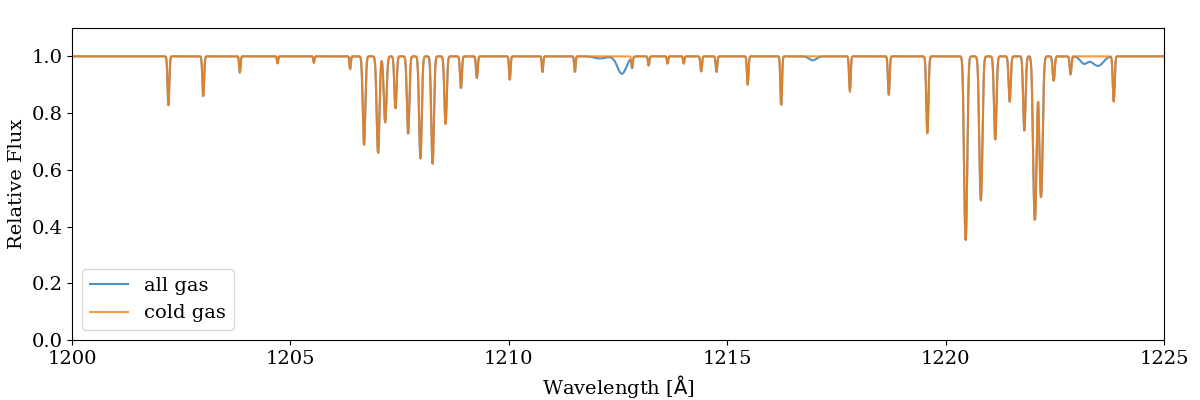In addition to generating a basic spectrum as demonstrated in the annotated example, the user can also customize the generated spectrum in a variety of ways. One can choose which spectral lines to deposit or choose different settings for the characteristics of the spectrograph, and more. The following code goes through the process of setting these properties and shows what impact it has on resulting spectra.

For this demonstation, we’ll be using a light ray passing through a very dense disk of gas, taken from the initial output from the AGORA isolated box simulation using ART-II in Kim et al. (2016). If you’d like to try to reproduce the spectra included below you can get the `LightRay` file from the Trident sample data using the command:

```\$ wget http://trident-project.org/data/sample_data/ART-II_ray.h5
```

Now, we’ll load up the ray using yt:

```import yt
import trident
```

## Setting the spectrograph

Let’s set the characteristics of the spectrograph we will use to create this spectrum. We can either choose the wavelength range and resolution and line spread function explicitly, or we can choose one of the preset instruments that come with Trident. To list the presets and their respective values, use this command:

```print(trident.valid_instruments)
```

Currently, we have three settings for the Cosmic Origins Spectrograph available: `COS-G130M`, `COS-G140L`, and `COS-G160M`, but we plan to add more instruments soon. To use one of them, we just use the name string in the `SpectrumGenerator` class:

```sg = trident.SpectrumGenerator('COS-G130M')
```

But instead, let’s just set our wavelength range manually from 1150 angstroms to 1250 angstroms with a resolution of 0.01 angstroms:

```sg = trident.SpectrumGenerator(lambda_min=1150, lambda_max=1250, dlambda=0.01)
```

From here, we can pass the ray to the `SpectrumGenerator` object to use in the construction of a spectrum.

## Choosing what absorption features to include

There is a `LineDatabase` class that controls which spectral lines you can add to your spectrum. Trident provides you with a default `LineDatabase` with 213 spectral lines commonly used in CGM and IGM studies, but you can create your own `LineDatabase` with different lines. To see a list of all the lines included in the default line list:

```ldb = trident.LineDatabase('lines.txt')
print(ldb)
```

which is reading lines from the ‘lines.txt’ file present in the data directory (see where is Trident installed?) We can specify any subset of these spectral lines to use when creating the spectrum from our master line list. So if you’re interested in just looking at neutral hydrogen lines in your spectrum, you can see what lines will be included with the command:

```print(ldb.parse_subset('H I'))
```

As a first pass, we’ll create a spectrum that just include lines produced by hydrogen:

```sg.make_spectrum(ray, lines=['H'])
sg.plot_spectrum('spec_H.png')
```

The resulting spectrum contains a nice, big Lyman-alpha feature.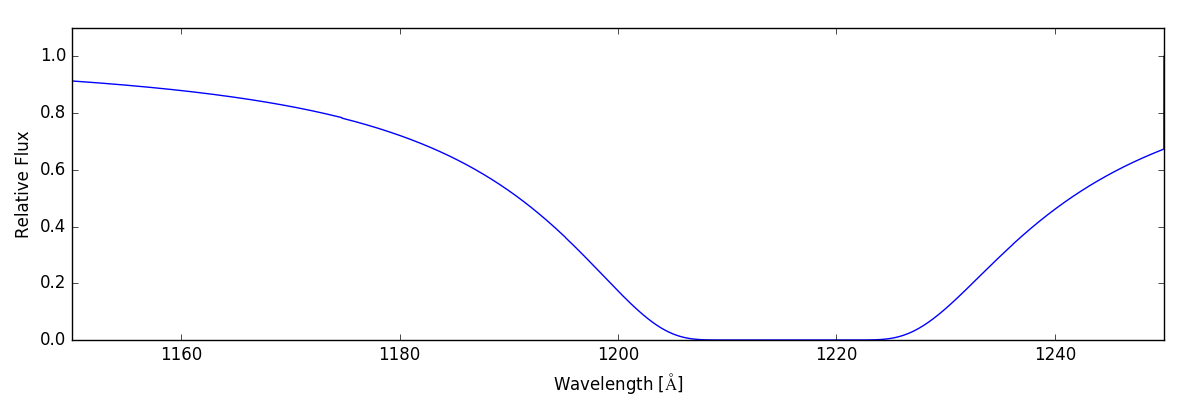If, instead, we want to shows the lines that would be in our spectral range due to carbon, nitrogen, and oxygen, we can do the following:

```sg.make_spectrum(ray, lines=['C', 'N', 'O'])
sg.plot_spectrum('spec_CNO.png')
```

And now we have: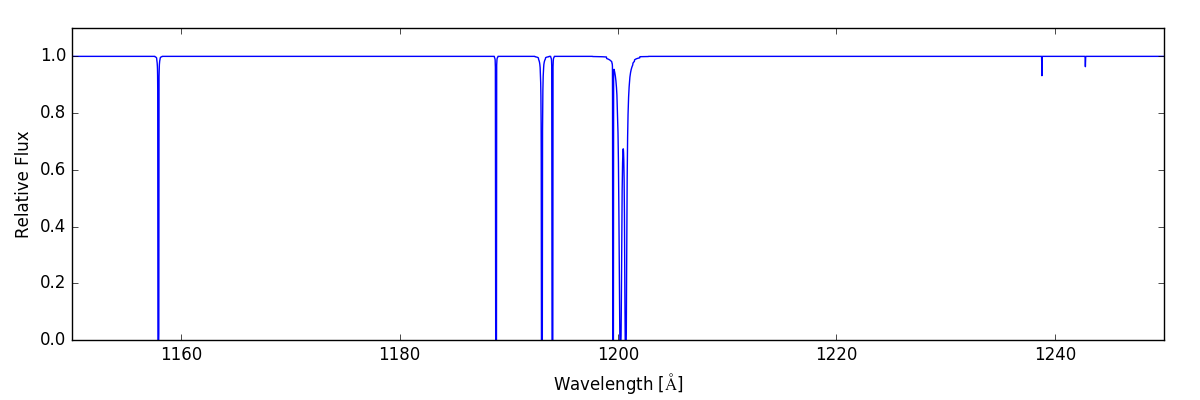We can see how these two spectra combined when we include all of the same lines:

```sg.make_spectrum(ray, lines=['H', 'C', 'N', 'O'])
sg.plot_spectrum('spec_HCNO.png')
```

which gives: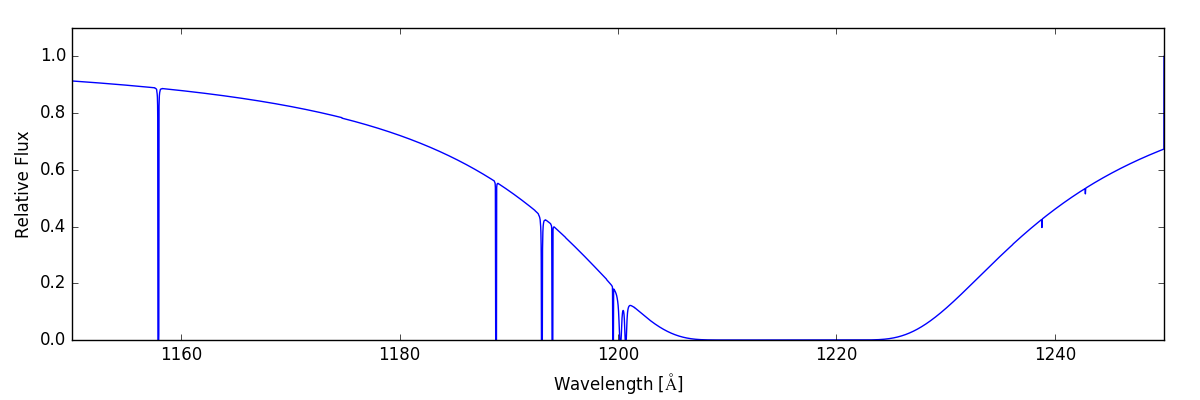We can get even more specific, by generating a spectrum that only contains lines due to a single ion species. For example, we might just want the lines from four-times-ionized nitrogen, N V:

```sg.make_spectrum(ray, lines=['N V'])
sg.plot_spectrum('spec_NV.png')
```

This spectrum only shows a couple of small lines on the right hand side.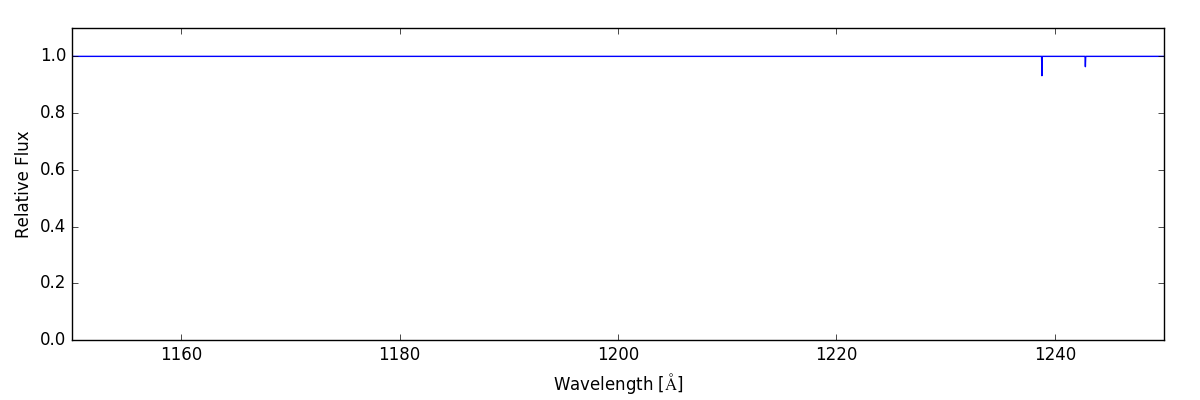But if that level of specificity isn’t enough, we can request individual lines:

```sg.make_spectrum(ray, lines=['C I 1193', 'C I 1194'])
sg.plot_spectrum('spec_CI_1193_1194.png')
```

And we end up with: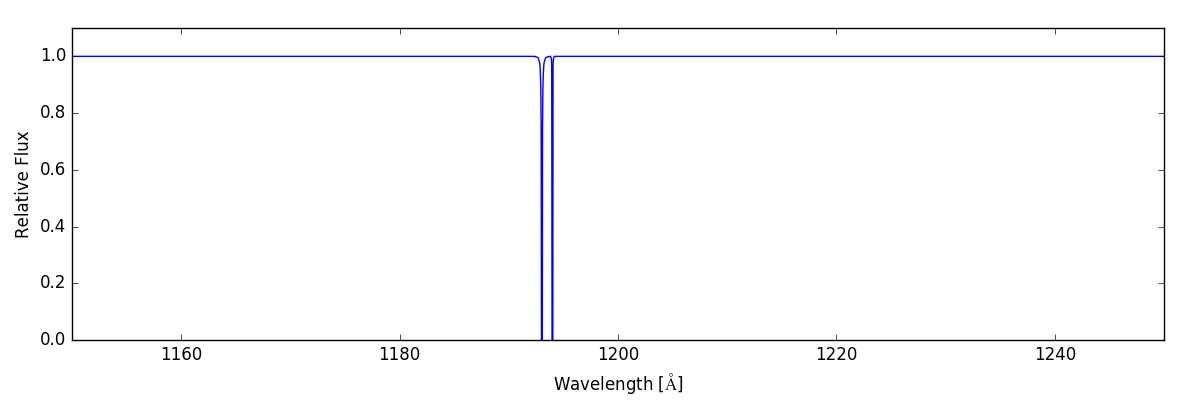Or we can just include all of the available lines in our `LineDatabase` with:

```sg.make_spectrum(ray, lines='all')
sg.plot_spectrum('spec_all.png')
```

Giving us: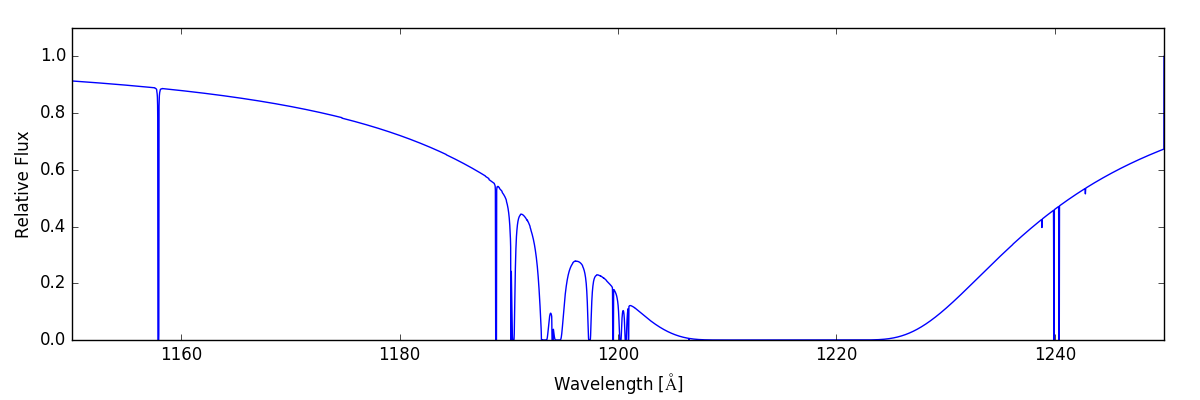To understand how to further customize your spectra, look at the documentation for the `SpectrumGenerator` and `LineDatabase` classes and other API documentation.

## Setting Wavelength Bounds Automatically

If you are interested in creating a spectrum that contains all possible absorption features for a given set of lines, the `SpectrumGenerator` can be configured to automatically enlarge the wavelength window until all absorption is captured. This is done by setting the `lambda_min` and `lambda_max` keywords to ‘auto’ and specifying a bin size with the `dlambda` keyword:

```sg = trident.SpectrumGenerator(lambda_min='auto', lambda_max='auto',
dlambda=0.01)
sg.make_spectrum("ray.h5", lines=['H I 1216'])
sg.plot_spectrum('spec_auto.png')
```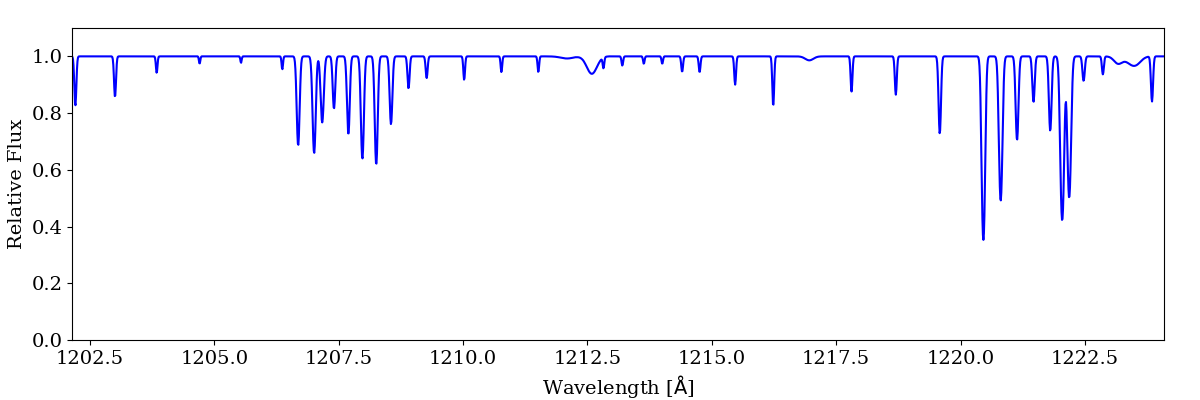Note, the above example is for a different ray than is used in the previous examples. The resulting spectrum will minimally contain all absorption present in the ray. This should be used with care when depositing multiple lines as this can lead to an extremely large spectrum.

## Making Spectra in Velocity Space

Trident can be configured to create spectra in velocity space instead of wavelength space where velocity corresponds to the velocity offset from the rest wavelength of a given line. This can be done by providing the keyword `bin_space='velocity'` to the `SpectrumGenerator`:

```sg = trident.SpectrumGenerator(lambda_min='auto', lambda_max='auto',
dlambda=1., bin_space='velocity')
sg.make_spectrum("ray.h5", lines=['H I 1216'])
sg.plot_spectrum('spec_velocity.png')
```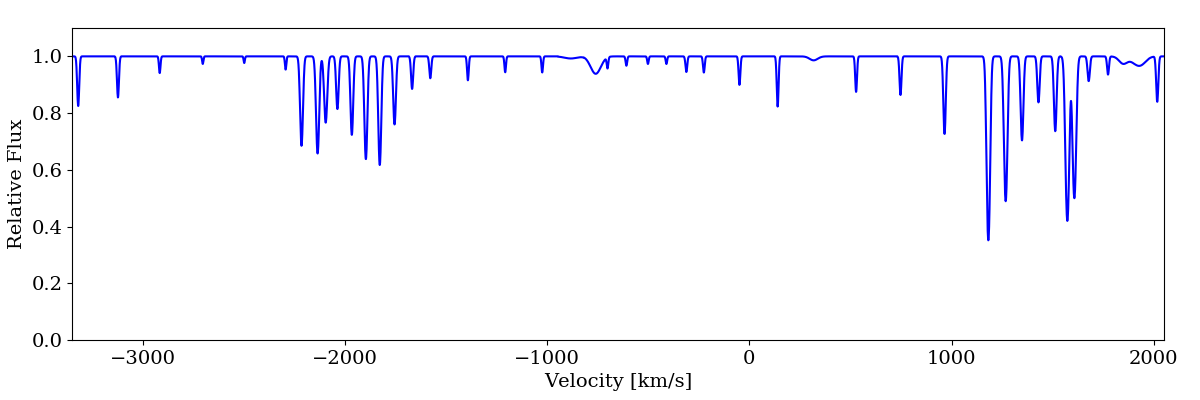When working in velocity space, limits and bin sizes should be provided in km/s. If more than one transition is added to the spectrum (e.g., Ly-a and Ly-b), the zero point will correspond to the rest wavelength of the first transition added.

## Making Spectra from a Subset of a Ray

The situation may arise where you want to see the spectrum that is generated by only a portion of the gas along a line of sight. For example, you may want to see the spectrum of only the cold gas. This can be done by creating a `YTCutRegion` from a loaded ray dataset:

```import trident
import yt

all_data = ds.all_data()
cold_gas = ds.cut_region(all_data, 'obj["gas", "temperature"] < 10000')

sg = trident.SpectrumGenerator(lambda_min=1200, lambda_max=1225,
dlambda=0.01)

# spectrum of entire ray
sg.make_spectrum(all_data, lines=['H I 1216'])
all_spectrum = sg.flux_field[:]

# spectrum of cold gas
sg.make_spectrum(cold_gas, lines=['H I 1216'])
cold_spectrum = sg.flux_field[:]

trident.plot_spectrum(sg.lambda_field, [all_spectrum, cold_spectrum],
label=['all gas', 'cold gas'], stagger=None)
```